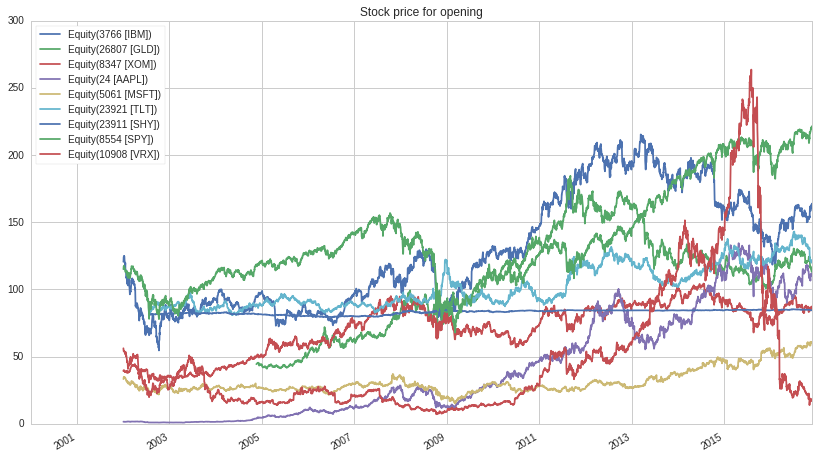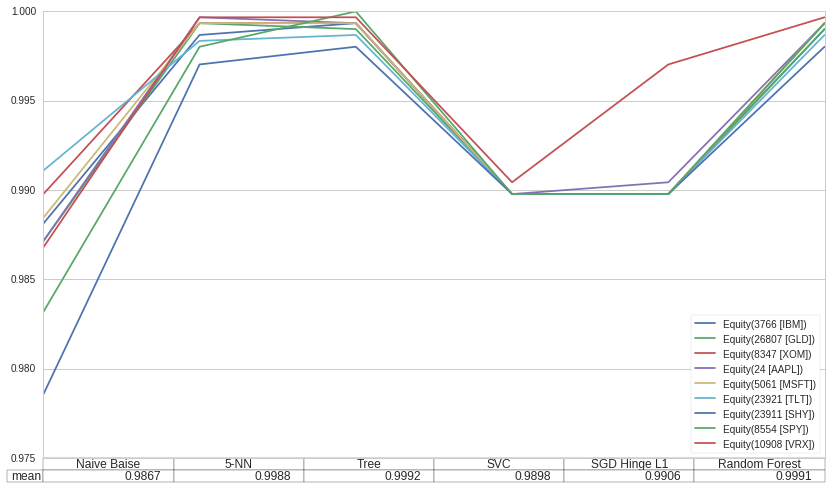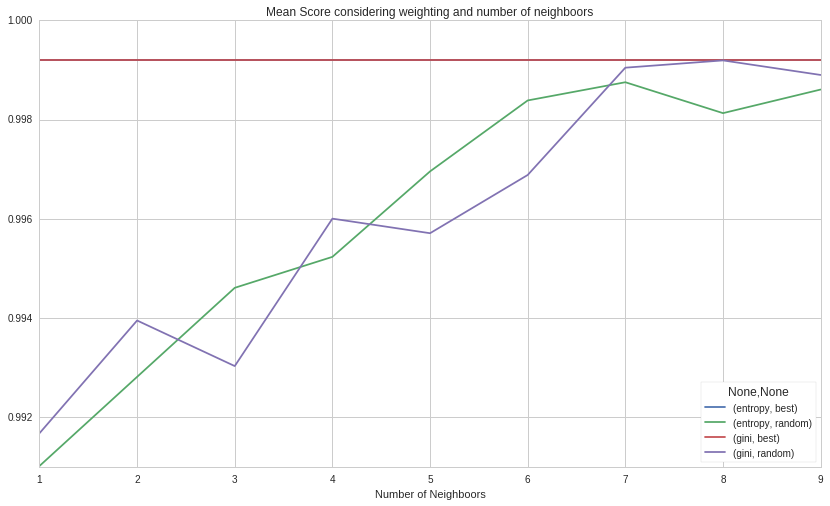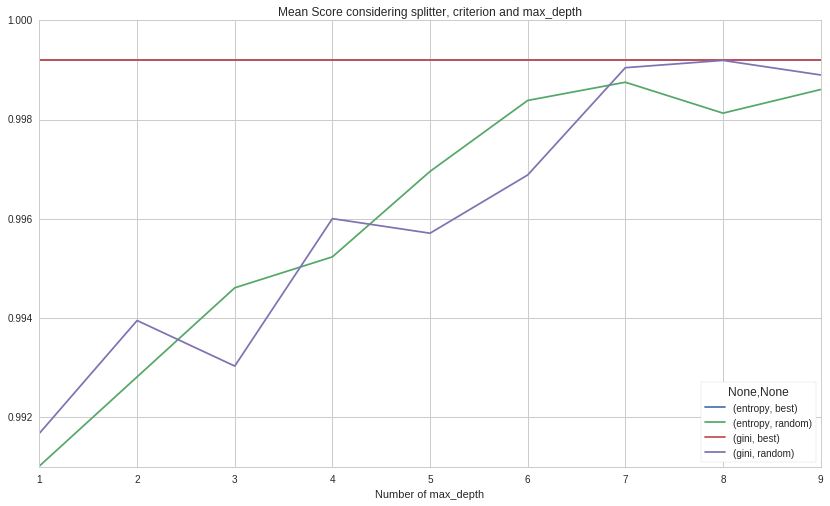Notebook

# Risk Management¶

## Determine the Value-at-Risk by using Machine Learning¶

I have listen to a lot of "Chat with Traders" lately and noticed, that a lot underlined the importance of good risk management. Basicly they don't hold any position above a defined maximal value (like some percantage of their booksize). But how to measure this threshold? Most of the interviewed traders are swing traders, that means they can't simply take the position size as "maximal to loose" since this can not applied to short positions.

I try to tackle this task here and will focus on the downside risk of long positions. However, the shown method can directly be applied to short positions. I am going to measure the risk for a holding period of one day.

This notebook contains four parts:

• Get the Data (Nine stocks with 17 years of historical data)
• Classifier Selection (Use different classifiers and compare their out-of-sample performance)
• Classifier Optimization (Parameter tweaking using cross-validation)

Here $\alpha$ is choosen as $0.01$, but different values were tested.

We will find that K-Nearest Neighboors and Decision Trees perform best and yield almost same results. I am planning to implement one of these as a pipeline factor. With that one can determine a maximal position size for each securtity.

In :
import numpy as np
import pandas as pd
import matplotlib.pyplot as plt

sec = ['IBM','GLD','XOM','AAPL','MSFT','TLT','SHY', 'SPY', 'VRX']

start_date='2000-01-01'
end_date='2016-11-30'

alpha = 0.01


## Get the Data¶

I work with a daily resolution and analyze the delta of open_price and low.

In :
data = get_pricing(sec, start_date=start_date, end_date=end_date, frequency='daily',
fields=['open_price', 'low'])

In :
data_log = np.log(data)
change = data_log['open_price'].subtract(data_log['low'])
change = change.dropna(axis=0)

data['open_price'].plot()
plt.title('Stock price for opening')

Out:
<matplotlib.text.Text at 0x7f6ccce79610>## Classifier Selection¶

Since the main task is to classify if a given change is tolerable or not (below the $1-\alpha$ quantile or not). We can use some well known classifiers like Nearest Neighboor, linear Regression, etc. We are going to choose some and compare their performance with a cross-validation.

In :
from sklearn.cross_validation import cross_val_score
from sklearn import tree, svm, neighbors, ensemble
from sklearn.linear_model import SGDClassifier
from sklearn.naive_bayes import GaussianNB
from sklearn.ensemble import RandomForestClassifier

def score(self, X, y):
y_pred = self.predict(X)
return (y_pred==y).mean()

def createXy(e):
X = change[e].values
pos = np.ceil(len(X)*alpha).astype(int)
y = X > np.sort(X, axis=0)[::-1][pos]
return X.reshape(-1, 1), y

clf = {'Tree': tree.DecisionTreeClassifier(),
'SGD Hinge L1': SGDClassifier(loss="hinge", penalty="l1"),
'5-NN': neighbors.KNeighborsClassifier(5),
'SVC': svm.SVC(),
'Naive Baise': GaussianNB(),
'Random Forest': RandomForestClassifier()}

scores_clf = pd.DataFrame(index=clf.keys(), columns=change.columns)
for el in change:
for key in clf:
X, y = createXy(el)
this_scores = cross_val_score(clf[key], X, y, cv=5, scoring=score)
scores_clf.loc[key, el] = this_scores.mean()


Let us look at the results, where the y-axis shows the mean-score (higher is better).

In :
table = pd.DataFrame(scores_clf.mean(axis=1).round(4), columns=['mean'], index=scores_clf.index).T

fig, ax = plt.subplots(1, 1)

ax.get_xaxis().set_visible(False)
scores_clf.plot(table=table, ax=ax)

Out:
<matplotlib.axes._subplots.AxesSubplot at 0x7f6cb4e050d0>The results differ for all securities, but clearly the most promising classifiers are 5-NN and Tree. This may be due to the fact, that we only have only one input dimension (which is quite easy and does not call for a more sophisticated approach).

## Classifier Optimization¶

To decide which of those two is better, we are going to tweak eachs parameter to obtain optimal results. However, one must be carefull to avoid overfitting/curvefitting.

### K-Nearest Neighboor¶

In the case of K-Nearest Neighboor there are only two parameters to choose:

1. Weighting (Constant or Inverse Distance)
2. Number of Neighboors $K=1,\dots$
In :
scores_par = pd.DataFrame(index=range(1,51), columns=['uniform', 'distance'])
for w in scores_par.columns:
for k in scores_par.index:
this_scores = []
clf = neighbors.KNeighborsClassifier(n_neighbors=k, weights=w)
for el in change.columns:
X, y = createXy(el)

this_scores.extend(cross_val_score(clf, X, y, scoring=score, cv=5))
scores_par.loc[k, w] = np.mean(this_scores)

In :
scores_par.plot()
plt.title('Mean Score considering weighting and number of neighboors')
plt.xlabel('Number of Neighboors')

Out:
<matplotlib.text.Text at 0x7f6cb4a73590>The inverse distanced used as weight function is clearly the better choice. For the number of neighboor we get, that less is better. In this certain instance One performed best. Since for larger $K$ the performance is almost the same, would tend to choose $K=5$ or larger to avoid problems with outliners in the data.

In :
criterions = ['gini', 'entropy']
splitters = ['random', 'best']
max_depths = np.arange(1, 10)

iter = ((criterion, splitter, max_depth) for criterion in criterions for splitter in splitters for max_depth in max_depths)

scores_par = {}
for (criterion, splitter, max_depth) in iter:
key = (criterion, splitter)
if not key in scores_par:
scores_par[key] = {}

this_scores = []
clf = tree.DecisionTreeClassifier(splitter=splitter, criterion=criterion, max_depth=max_depth)
for el in change.columns:
X, y = createXy(el)

this_scores.extend(cross_val_score(clf, X, y, scoring=score, cv=5))
scores_par[key][max_depth] = np.mean(this_scores)

scores_par = pd.DataFrame(scores_par)

In :
scores_par.plot()
plt.title('Mean Score considering splitter, criterion and max_depth')
plt.xlabel('Number of max_depth')

Out:
<matplotlib.text.Text at 0x7f6cb48c6310>As one can see, the criterion and max_depth are irrelevant as long as splitter is chosen as "best".